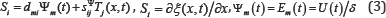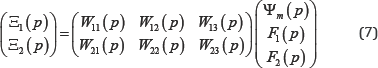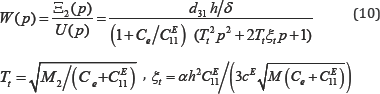• Submissions# Electro magneto Elastic Actuator for Nanotechnology and Biotechnology

Afonin SM*

Department of Intellectual Technical Systems, National Research University of Electronic Technology (MIET), Russia

*Corresponding author: Afonin SM, Department of Intellectual Technical Systems, National Research University of Electronic Technology (MIET),Russia

Submission: November 7, 2017 ; Published: January 05, 2018

DOI: 10.31031/MAPP.2018.01.000510ISSN 2637-7756
Volume1 Issue2

#### Abstract

The electro magneto elastic actuator is used for precise alignment in the nanotechnology, the biotechnology, the adaptive optics. The structural- parametric models, the solution of wave equation, the generalized matrix transfer function of the electro magneto elastic actuator are determined. The dynamic and static characteristics of the actuator are obtained. The parametric structural schematic diagram and the transfer functions are determined for the control systems in the nanotechnology and the biotechnology. The structural-parametric models of the piezoactuator for the transverse, longitudinal, shift piezoelectric effects are constructed.

Keywords: Electro magneto elastic actuator; Piezoactuator; Structural parametric model; Parametric structural schematic diagram; Transfer function

#### Introduction

The electro magneto elastic actuator of the piezoeffect, the piezomagnetic effect, the electrostriction or the magnetostriction effect is used for precise alignment in the nanotechnology, the biotechnology and the adaptive optics [1-32]. The piezoactuator on the inverse piezoeffect is serves for the actuation of mechanisms or the management, converts electrical signals into displacement and force [1-8]. The piezoactuator for the nanomechanics is provided the displacement from nanometers to tens of micrometers, a force to 1000N. The piezoactuator is used for research in the nanomedicine and the nanobiotechnology for the scanning tunneling microscopes, scanning force microscopes and atomic force microscopes [14-32].

In the present paper the generalized structural-parametric model and the generalized parametric structural schematic diagram of the electro magneto elastic actuator are constructed by solving the wave equation with the Laplace transform for the equation of the electro magnetolasticity, the boundary conditions on loaded working surfaces of the actuator, the strains along the coordinate axes. The transfer functions and the parametric structural schematic diagrams of the piezoactuator are obtained from the generalized structural-parametric model. In [6,7] was determined the solution of the wave equation of the piezoactuator. In the [14-16,30,31] were obtained the structural-parametric models, the schematic diagrams for simple piezoactuator and were transformed to the structural-parametric model of the electro magneto elastic actuator. The structural-parametric model of the electroelastic actuator was determined in contrast electrical equivalent circuit for calculation of piezoelectric transmitter and receiver [9-12]. In [8,27] was used the transfer functions of the piezoactuator for the decision problem absolute stability conditions for a system controlling the deformation of the electro magneto elastic actuator. The elastic compliances and the mechanical and adjusting characteristics of the piezoactuator were found in [18,21-23,28,29] for calculation its transfer functions and the structural-parametric models. The structural-parametric model of the multilayer and compound piezoactuator was determined in [18-20]. In this paper is solving the problem of building the generalized structural parametric model and the generalized parametric structural schematic diagram of the electro magneto elastic actuator for the equation of electro magneto elasticity.

#### Structural-Parametric Model of Electro magneto Elastic Actuator

The general structural-parametric model and the parametric structural schematic diagram of the electro magneto elastic actuator are obtained. In the electro elastic actuator are presented six stress components Ti, T, T3, T4,T,T6, the components Ti - T3 are related to extension-compression stresses, T4 - T to shear stresses. For the electro elastic actuator its deformation corresponds to stressed state. For polarized piezoceramics PZT the matrix state equations [12,14] connected the electric and elastic variables have the form two equations, then the first equation describes the direct piezoelectric effect, the second - the inverse piezoelectric effect.Where D is the column matrix of electric induction; S is the column matrix of relative deformations; T is the column matrix of mechanical stresses; E is the column matrix of electric field strength; sE is the elastic compliance matrix for E = const; εT is the matrix of dielectric constants for T= const; dt is the transposed matrix of the piezoelectric modules.

The piezoactuator (piezoplate) has the following properties: δ- the thickness, h - the height,b - the width, respectively i = { S, h, b the length of the piezoactuator for the longitudinal, transverse and shift piezoeffect. The direction of the polarization axis P, i.e., the direction along which polarization was performed, is usually taken as the direction of axis 3. The equation of the inverse piezo effect for controlling voltage [6,12] has the form.where is the relative displacement of the cross section of the piezoactuator along axis i, Ψm (t) is the control parameter along axis m, dmi is the piezomodule,Em (t) is the electric field strength along axis m, U(t) is the voltage between the electrodes of actuator,sijΨ is the elastic compliance, Tjis the mechanical stress along axis j and i, j = 1, 2, ..., 6; m= 1, 2, 3. The main size for the piezoactuator, respectively, the thickness, the height, the width for the longitudinal, transverse, shift piezoeffect. For calculation of actuator is used the wave equation [6,7,12,14] for the wave propagation in a long line with damping but without distortions. After Laplace transform is obtained the linear ordinary second-order differential equation with the parameter p, whereupon the original problem for the partial differential hyperbolic equation of type using the Laplace transform is reduced to the simpler problem [6,13] for the linear ordinary differential equation.where( x, p) is the Laplace transform of the displacement of the section of the piezoactuator, γ = p/cΨ + α is the propagation coefficient, cΨ is the sound speed for * = const ,is the damping coefficient, Ψ is the control parameter: E for the voltage control, D for the current control, H for the magnet field strength control. From (3, 5), the boundary conditions on loaded surfaces, the strains along the axes the system of equations for the generalized structural-parametric model and the generalized parametric structural schematic diagram Figure 1 of the actuator are determinedFigure 1: Generalized parametric structural schematic diagram of the electro magneto elastic actuator.Is the coefficient of the electro magnetolasticity (piezomodule or coefficient of magnetostriction). Figure 1 shows the generalized parametric structural schematic diagram of the electro magneto elastic actuator corresponding to the set of equations (6). The generalized transfer functions of the electro magneto elastic actuator are the ratio of the Laplace transform of the displacement of the face actuator and the Laplace transform of the corresponding control parameter or the force at zero initial conditions. From (6) the generalized matrix equation of the transfer functions of electro magneto elastic actuator.For and the static displacement of the faces of the piezoactuator for the transverse piezoeffect are obtained.For the piezoactuator from PZT under the transverse piezoeffect at m <1 and m << M2, d31 = 2.5-10-13°m/V,h/δ = 20 ,U = 100 V,M1 = 2 kg andM2, = 8 kg the static displacement of the faces are determined ξ1(∞) = 400 nm, ξ2(∞)= lOOnm, ξ1(∞)+ξ2(∞) = 500 nm. For the approximation of the hyperbolic cotangent by two terms of the power series in transfer function (7) the following expressions of the transfer function of the piezoactuator is obtained for the elastic-inertial load at M1→ ∞, m << M2 under the transverse piezoeffectWhere U(p) the Laplace is transform of the voltage, Tt is the time constant and ξt is the damping coefficient of the piezoactuator. The expression for the transient response of the voltage-controlled piezoactuator for the elastic-inertial load under the transverse piezoeffect is determined.Where ξmthe steady-state value of displacement of the piezoactuator is,um is the amplitude of the voltage. For the voltage-controlled piezoactuator from the piezoceramics PZT under the transverse piezoelectric effect for the elastic-inertial load M1 →(∞), m << M2 and input voltage with amplitude Um = 120 V at d31 = 2.5-10-10m/V,h/δ = 20,M2 =4kg, cE11 = 2.107 N/m,ce =0.5-107 H/m are obtained values ξm=480 nm, Tt = 0.4.1 10-3c.

#### Results and Discussions

The structural-parametric model and parametric structural schematic diagrams of the voltage-controlled piezoactuator for the longitudinal, transverse and shift piezoelectric effects are determined from the generalized structural-parametric model of the electro magneto elastic actuator for the nanotechnology and the biotechnology with the replacement of the generalized parameters. The generalized structural-parametric model, the generalized parametric structural schematic diagram and the matrix equation of the electro magneto elastic actuator are obtained from the solutions of the wave equation with the Laplace transform and from its deformations along the coordinate axes. From the generalized matrix equation for the transfer functions of the electro magneto elastic actuator after algebraic transformations are constructed the matrix equations of the piezoactuator for the longitudinal, transverse and shift piezoelectric effects.

#### Conclusion

The deformations of the actuator are described by the matrix equation for the transfer functions of the electro magneto elastic actuator for precise alignment in the nanotechnology, the biotechnology, the adaptive optics. The structural-parametric model, the matrix transfer function of the electro magneto elastic actuator is determined. For the control systems in the nanotechnology and the biotechnology. The structural-parametric model, the matrix equation and the parametric structural schematic diagram of the piezoactuator for the transverse, longitudinal, shift piezoelectric effects are obtained from the generalized structural- parametric model of the electro magneto elastic actuator. From the solution of the wave equation, from the equation of the electro magneto elasticity and the deformations along the coordinate axes the generalized structural-parametric model and the generalized parametric structural schematic diagram of the electro magneto elastic actuator are constructed for the control systems for the nanotechnology and the biotechnology.

We at Crimson Publishing are a group of people with a combined passion for science and research, who wants to bring to the world a unified platform where all scientific know-how is available read more...